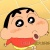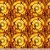0+0+0+0+0+0+0+0+0+0+0+0+0+0+0+0+0+0+0+0+0+0+0+0+0+0+0+0+0+0+0+0+0+Deep learning frameworks leverage GPUs to perform massively-parallel computations over batches of many training examples efficiently. However, for certain tasks, one may be interested in performing per-example computations, for instance using per-example gradients to evaluate a quantity of interest unique to each example. One notable application comes from the field of differential privacy, where per-example gradients must be norm-bounded in order to limit the impact of each example on the aggregated batch gradient. In this work, we discuss how per-example gradients can be efficiently computed in convolutional neural networks (CNNs). We compare existing strategies by performing a few steps of differentially-private training on CNNs of varying sizes. We also introduce a new strategy for per-example gradient calculation, which is shown to be advantageous depending on the model architecture and how the model is trained. This is a first step in making differentially-private training of CNNs practical.0+0+0+下载预览We present the Hue-Net - a novel Deep Learning framework for Intensity-based Image-to-Image Translation. The key idea is a new technique termed network augmentation which allows a differentiable construction of intensity histograms from images. We further introduce differentiable representations of (1D) cyclic and joint (2D) histograms and use them for defining loss functions based on cyclic Earth Mover's Distance (EMD) and Mutual Information (MI). While the Hue-Net can be applied to several image-to-image translation tasks, we choose to demonstrate its strength on color transfer problems, where the aim is to paint a source image with the colors of a different target image. Note that the desired output image does not exist and therefore cannot be used for supervised pixel-to-pixel learning. This is accomplished by using the HSV color-space and defining an intensity-based loss that is built on the EMD between the cyclic hue histograms of the output and the target images. To enforce color-free similarity between the source and the output images, we define a semantic-based loss by a differentiable approximation of the MI of these images. The incorporation of histogram loss functions in addition to an adversarial loss enables the construction of semantically meaningful and realistic images. Promising results are presented for different datasets.0+0+0+下载预览Crowd counting problem that counts the number of people in an image has been extensively studied in recent years. In this paper, we introduce a new variant of crowd counting problem, namely "Categorized Crowd Counting", that counts the number of people sitting and standing in a given image. Categorized crowd counting has many real-world applications such as crowd monitoring, customer service, and resource management. The major challenges in categorized crowd counting come from high occlusion, perspective distortion and the seemingly identical upper body posture of sitting and standing persons. Existing density map based approaches perform well to approximate a large crowd, but lose important local information necessary for categorization. On the other hand, traditional detection-based approaches perform poorly in occluded environments, especially when the crowd size gets bigger. Hence, to solve the categorized crowd counting problem, we develop a novel attention-based deep learning framework that addresses the above limitations. In particular, our approach works in three phases: i) We first generate basic detection based sitting and standing density maps to capture the local information; ii) Then, we generate a crowd counting based density map as global counting feature; iii) Finally, we have a cross-branch segregating refinement phase that splits the crowd density map into final sitting and standing density maps using attention mechanism. Extensive experiments show the efficacy of our approach in solving the categorized crowd counting problem.0+0+0+下载预览0+0+0+
Top